# 14 Spheres

The set of points in space whose distance to a fixed point (the center) is a fixed positive number (the radius) is a sphere. A circle of radius r and center (x,y,z) has equation

(x-x)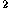+(y-y)+(z-z)=r,

or

x+y+z-2xx-2yy-2zz+x+y+z-r=0.

Conversely, an equation of the form

x+y+z+2dx+2ey+2fz+g=0

defines a sphere if d+e+f>g; the center is (-d, -e, -f) and the radius is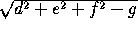.

Four points not on the same plane determine a unique sphere. If the points have coordinates (x,y,z), (x,y,z), (x,y,z) and (x,x,z), the equation of the sphere is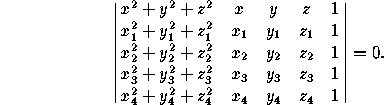Given two points P=(x,y,z) and P=(x,y,z), there is a unique sphere whose diameter is PP; its equation is

(x-x)(x-x)+(y-y)(y-y)+(z-z)(z-z)=0.

The area of a sphere of radius r is 4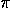r, and the volume isr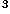.

The area of a spherical polygon (that is, of a polygon on the sphere whose sides are arcs of great circles) is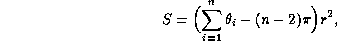where r is the radius of the sphere, n is the number of vertices, and the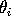are the internal angles of the polygons in radians. In particular, the sum of the angles of a spherical triangle is always greater than=180°, and the excess is proportional to the area.

### Spherical cap

Let the radius be r (Figure 1, left). The area of the curved region is 2rh=p. The volume of the cap ish(3r-h)=h(3a+h).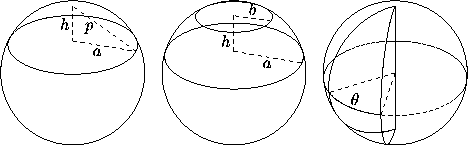Figure 1: Left: a spherical cap. Middle: a spherical zone (of two bases). Right: a spherical segment.

### Spherical zone (of two bases)

Let the radius be r (Figure 1, middle). The area of the curved region is 2rh. The volume of the zone ish(3a+3b+h).

### Spherical segment and lune

Let the radius be r (Figure 1, right). The area of the curved region (lune) is 2r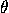, the angle being measured in radians. The volume of the segment isr.

Next: 15 Surfaces of Revolution. The Torus
Up: Part II: Three-Dimensional Geometry
Previous: 13.3 ConesThe Geometry Center Home Page

Silvio Levy
Wed Oct 4 16:41:25 PDT 1995

This document is excerpted from the 30th Edition of the CRC Standard Mathematical Tables and Formulas (CRC Press). Unauthorized duplication is forbidden.